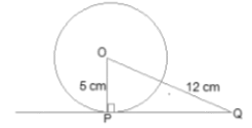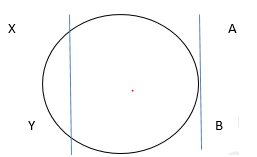# NCERT Solutions for Class 10 Maths Chapter 10:Circle Exercise 10.1

In this page we have NCERT Solutions for Class 10 Maths Chapter 10:Circle for EXERCISE 10.1 . Hope you like them and do not forget to like , social share and comment at the end of the page.
Points to Remember
• The tangent to a circle is a special case of the secant, when the two end  points of its  corresponding chord coincide.
• The common point of the tangent and the circle is called the point of contact
• The tangent at any point of a circle is perpendicular to the radius through the point of contact
Question 1.;
How many tangents can a circle have?
A circle can have infinite tangents.
Question 2.
Fill in the blanks:
(i) A tangent to a circle intersects it in ............... point(s).
(ii) A line intersecting a circle in two points is called a .............
(iii) A circle can have ............... parallel tangents at the most.
(iv) The common point of a tangent to a circle and the circle is called ............
(i) one
(ii) secant
(iii) two
(iv) point of contact

Question 3.
A tangent PQ at a point P of a circle of radius 5 cm meets a line through the centre O at a point Q so that OQ = 12 cm. Length PQ is :
(A) 12 cm
(B) 13 cm
(C) 8.5 cm
(D) √119cmWe know that the line drawn from the centre of the circle to the tangent is perpendicular to the tangent.
So OP ⊥ PQ
Now By Pythagoras theorem in ΔOPQ,
OQ2 = OP2 + PQ2
(12)= 52 + PQ2
PQ2 = 119
PQ = √119 cm
(D) is the correct option.

Question 4.
Draw a circle and two lines parallel to a given line such that one is a tangent and the other, a secant to the circle.AB and XY are two parallel lines where AB is the tangent to the circle at point C while XY is the secant to the circle.Go back to Class 10 Main Page using below links

### Practice Question

Question 1 What is $1 - \sqrt {3}$ ?
A) Non terminating repeating
B) Non terminating non repeating
C) Terminating
D) None of the above
Question 2 The volume of the largest right circular cone that can be cut out from a cube of edge 4.2 cm is?
A) 19.4 cm3
B) 12 cm3
C) 78.6 cm3
D) 58.2 cm3
Question 3 The sum of the first three terms of an AP is 33. If the product of the first and the third term exceeds the second term by 29, the AP is ?
A) 2 ,21,11
B) 1,10,19
C) -1 ,8,17
D) 2 ,11,20